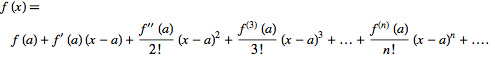# More Taylors expansion

• Gwilim

#### Gwilim

I still need help with my first Taylors expansion thread, but this is a separate problem

## Homework Statement

a) Give the formula for the Taylor expansion of the function f(x) about the point x=a. You should include the formula for the coeffiecients of the expansion in terms of the derivatives of f(x)
b) Find the first three non-zero terms of the Taylor expansion about x=1 of the function
f(x)=exp(x^3-1)

2. The attempt at a solution

a)
f(x + a) = f(a) + xf'(a) + x^2f''(a)/2! + ... + x^nf^n(a)/n! ... where f^n(a) means the nth derivative of f(a)

I think I might have missed the point of this question.

b)
f(x+1) = 1 + 3x^3.e^(3x-1) + [9x^6.e^(3x-1)+6x^3.e^(3x-1)]/2

I'm assuming here that exp(x^3-1) means e^(x^3-1), but this isn't notation that's used anywhere else on the paper.

Taylor expansion about x = a by definition:

f(x) = f(a) + (x-a)*f'(a) + (x-a)^2/2!*f''(a) + ...

Use the formula: